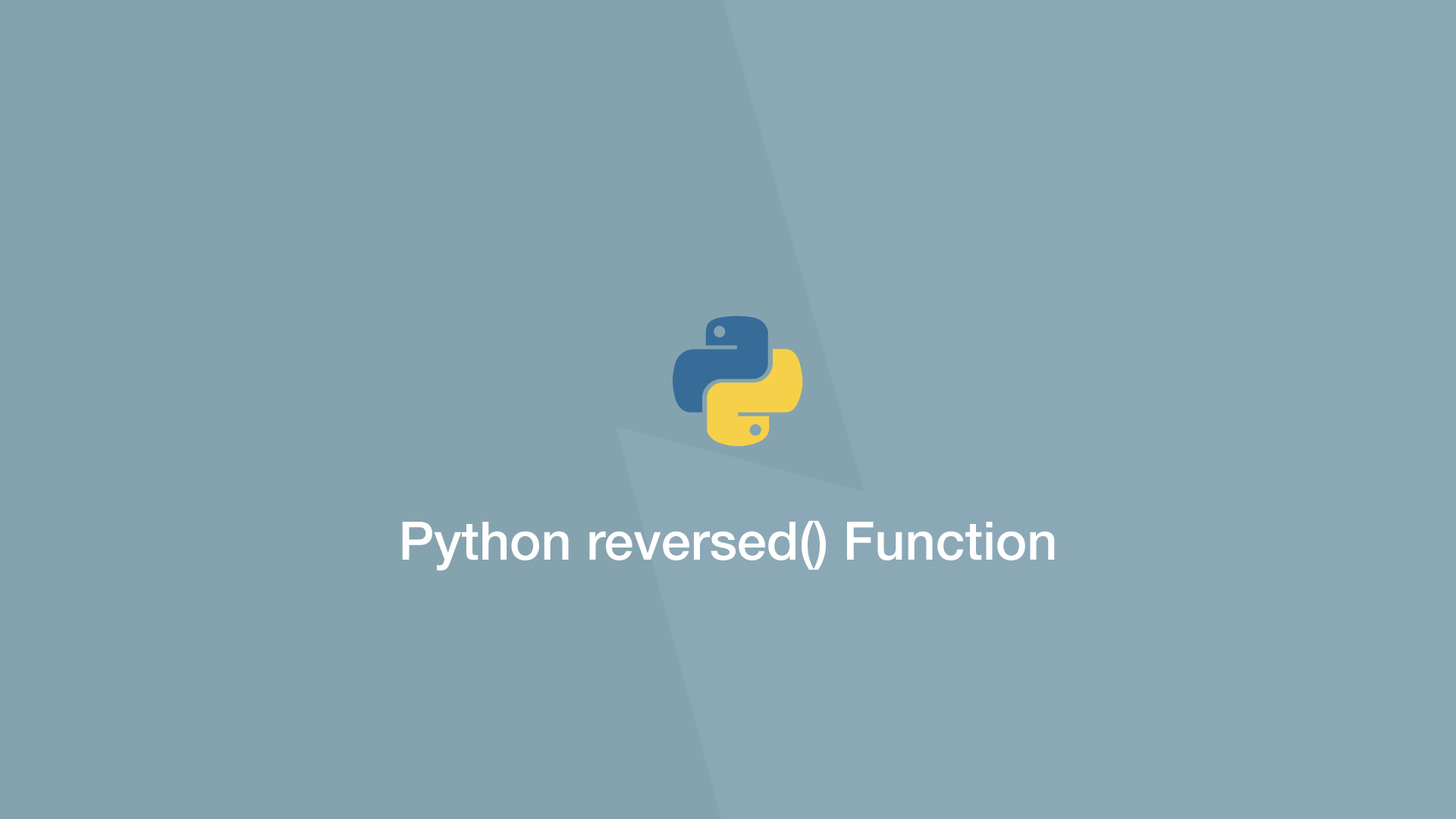# How to use the Python reversed() FunctionThe `reversed()` function in Python takes a sequence and reverses its order. The first element will become the last, the second becomes the second to last .etc.

## reversed() Syntax

The `reversed()` function accepts one argument; the sequence to reverse, which could be a string, list, tuple .etc.

``````reversed(sequence)
``````

## Reverse a String in Python

Let's demonstrate how this function works by reversing a string.

``````string = 'hello'

result = reversed(string)

print(result)
``````
``````<reversed object at 0x7fd5bf654250>
``````

Just using the `reversed()` function on its own, we get a `reversed object` back. To get a string back, we also need to use Python `.join()` function like this:

``````string = 'hello'

result = ''.join(reversed(string))

print(result)
``````
``````olleh
``````

Note`reversed()` does not modify the original sequence.

## Reverse a List in Python

Here is another example, this time reversing the order of a list. To convert the `reversed object` back to a list, we will use the Python `list()` function.

``````items = ['orange', 'apple', 'strawberry']

result = list(reversed(items))

print(result)
``````
``````['strawberry', 'apple', 'orange']
``````

#### Related Tutorials### How to Create and Use Lists (Arrays) in Python

September 01, 2020### How to Sort Dictionaries by Value In Python

September 22, 2020April 10, 2021